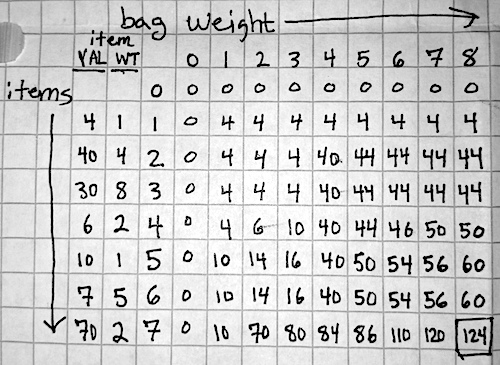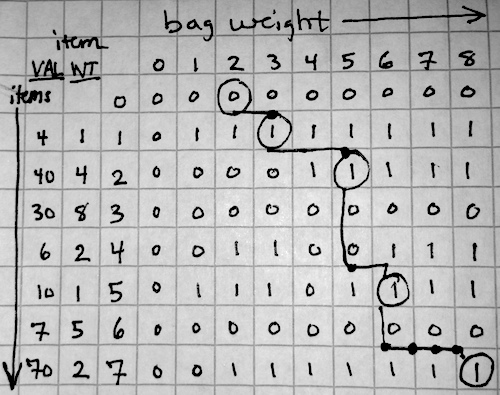## The Knapsack Problem

You run an import-export company and are packing for a trip. You are allowed one bag of fixed size, and you have more items than can fit in the bag that you wish to bring back with you in order to sell. How can you maximize the value of the items that you fit in the bag? How can we maximize a given quantity subject to a constraint?

## Example Problem

``````const bagSize = 8;
let items = [
{name: 'cat dish', value: 4, size: 1},
{name: 'laptop', value: 40, size: 4},
{name: 'Boxed Set of Knuth', value: 30, size: 8},
{name: 'assorted snacks', value: 6, size: 2},
{name: 'catnip', value: 10, size: 1},
{name: 'clothing', value: 7, size: 5},
{name: 'diamonds', value: 70, size: 2}
];
let optimalBagContents = knapsackSolver(items, bagSize); // ??
``````

Let’s write our `knapsackSolver` function.

A naive approach would be to loop over the items and add them to the bag so long as they fit, without considering their values, until the bag is full:

``````function knapsackSolver(items, size) {
let res = [];
let currentSz = 0;
while (currentSz < size && items.length) {
let newItem = items.shift();
if (newItem.size+currentSz<=size) {
currentSz+=newItem.size;
res.push(newItem);
}
}
return {contents: res, value: res.reduce((acc,b)=>acc+=b.value,0)};
}
``````

This gives us

``````​​​​​​​​​​{ contents: ​​​​​
​​​​​   [ { name: 'cat dish', value: 4, size: 1 },​​​​​
​​​​​     { name: 'laptop', value: 40, size: 4 },​​​​​
​​​​​     { name: 'assorted snacks', value: 6, size: 2 },​​​​​
​​​​​     { name: 'catnip', value: 10, size: 1 } ],​​​​​
​​​​​  value: 60 }​​​​​
``````

A value of 60 is not bad. But we can see just by looking at the items that we can clearly do better. Filling up the bag too early caused us to miss out on that small bag of diamonds worth 70. If we took diamonds + laptop + catnip + cat dish (70 + 40 + 10 + 4), we’d be much better off, at a value of 124.

So there’s the question: how can we make our code figure out what, in this basic case, was easy for us to calculate by hand?

## Solving the Problem

A naive solution would be to generate all possible combinations of the given items, calculate each combination’s value, and return the maximum.

To do this, we’d need to calculate the Power Set of the items, which is the set of all subsets of the items. This makes sense: if we are naively grouping the items and checking all possible combinations, we’re not excluding cases where the pack isn’t full. So, we have one group with each item individually. One group with item one and item two, item one and item three, item one and item four, etc. etc.

The Power Set is on the order of 2^n, or an exponential runtime. This is completely prohibitive for solving any but the most trivial of problems. It’s not uncommon to have a set of 100 items you need to maximize. Assuming those items were merely 1 byte each (numbers between 0-255), the operation would take 2^30 bytes, or just over a gigabyte of memory.

Clearly we need a way to generate combinations relative to the constraints as we construct the solution, not after the fact. Dynamic Programming is a paradigm that allows us to do this: we can use the solution to previously calculated problems to dictate the space over which we search for the next steps in the solution.

How does this apply to the knapsack problem?

At a high level, how do we go about filling the knapsack? We iterate through the items and look at the current state of the knapsack. We ask these questions:

1. Will the item fit? If not, skip it. Add the previous row’s value, since that’s the highest value we can attain.
2. If the item fits, we decide whether or not to add it by comparing two values:

A) The value of not adding the item at all (the value of the row immediately above).

B) The value of adding the item and then the highest-valued-combination-of-items of size (current knapsack size - potential new item size). For example, if the size of the new item is 1, and we’re currently considering a pack of size 3, we look up the highest-value combination of size 2.

We can find the highest-value combination by going up one row and back `size` columns.

If value A > value B, we don’t add the item and copy down the value of the row above.

If value B > value A, we add the item and record the combined value in the cell.

The beauty of this algorithm is that we assemble the answers we need to determine whether or not to add an item as we iterate through the items. When we finish filling out the array, we’ll have our answer of the maximum value: it’s just the `[last row][last column]`.

There are a few relations that make this algorithm work that you can find here on the Wikipedia.

Straight from the wiki:

• m[i, w] is the maximum value using i items for a given weight.
• m[0, w] is zero for all w, since zero items have zero value.
• m[i, w] if the new item doesn’t fit: m[i-1, w].
• If the new item fits: max(m[i-1, w], m[i-1, w-newItem weight] + valueNewItem)

## The Nuts and Bolts

We’ll solve the problem by creating a grid with a width equal to the weight the knapsack can hold, and a height equal to the number of items we have to put in the sack.

When the knapsack is full and we’ve run through all of our items, the maximum value will be the last row and last column of the array.

We track whether or not we added an item to the knapsack with an auxiliary array: 1 indicates we picked it up, and 0 indicates that we did not pick it up. We can use this auxiliary array `keepArr` after the fact to create a packing list of items that constitute the maximum value for a given weight.

``````const bagSize = 8;
let items = [
{name: 'cat dish', value: 4, size: 1},
{name: 'laptop', value: 40, size: 4},
{name: 'Boxed Set of Knuth', value: 30, size: 8},
{name: 'assorted snacks', value: 6, size: 2},
{name: 'catnip', value: 10, size: 1},
{name: 'clothing', value: 7, size: 5},
{name: 'diamonds', value: 70, size: 2}
];

let values = [0, ...items.map(e=>e.value)];
let weights = [0, ...items.map(e=>e.size)];
const N = items.length;

const arrayGen = (m, n) => {
let M = new Array(bagSize+1);
M=new Array(bagSize+1).fill(0);
for (var i=1;i<=N;i++) { M[i] = new Array(bagSize); }
return M;
}

let M = arrayGen(bagSize, N);
let keepArr = arrayGen(bagSize, N);

for (var i=1; i<=N; i++) {
for (var j=0; j<=bagSize; j++) {
if (weights[i] > j) { // we can't add
M[i][j] = M[i-1][j];
keepArr[i][j]=0; // we can't keep this item
}
else {
const valueIfLeft = M[i-1][j];
const valueIfTaken = M[i-1][j-weights[i]] + values[i];
if (valueIfTaken >= valueIfLeft) { // maximize the value
M[i][j] = valueIfTaken;
keepArr[i][j]=1; // we can keep this item
} else {
M[i][j] = valueIfLeft;
keepArr[i][j]=0;
}
}
}
}
``````### Walking Through The Code

The code makes a lot more sense if you look at this example grid. We construct an array that is 1-based (so we fill out the zero-th index with a 0 and never use it). This makes the array indexing much easier, since we don’t have to worry about index-1 being out of bounds.

Then, we list the number of items as rows and the integer weight values as columns.

Note each row in the image corresponds to the `items` array above. We’ve got the cat dish at the top and the diamonds at the bottom.

So let’s walk through the diamonds row. Keep in mind, each time we say “is bigger than”, we’re walking up one row (the previous item) and back 2 (the diamonds’ weight).

• [7,0], the value is zero, because there’s no item in a bag with zero weight.
• [7,1], the value is 10, because we can’t fit the diamonds (size 2) in a bag of size 1.
• [7,2], the value is 70, because 70 + 0 is bigger than 14.
• [7,3], the value is 80, because 70 + 10 is bigger than 16.
• [7,4], the value is 84, because 70 + 14 is bigger than 40.
• [7,5], the value is 86, because 70 + 16 is bigger than 50.
• [7,6], the value is 110, because 70 + 40 is bigger than 54.
• [7,7], the value is 120, because 70 + 50 is bigger than 56.
• [7,8], the value is 124, because 70 + 54 is bigger than 60.

### Figuring Out Which Items Constitute The Max Value

``````let keptElements=[];
let eleKey = bagSize;
for (var i=N; i>0; i--) {
if (keepArr[i][eleKey]==1) {
keptElements.push(i-1);
eleKey -= weights[i];
}
}
``````##### Traversing the Keep Array

All we have to do to figure out which elements constitute the highest value is to traverse our keepArray, starting at the solution point. If the value is 1, we add that element’s index (minus one, since our matrix is 1-based) to the results array, and then we decrement our weight index by the weight of that element.

``````​​​​​[ { name: 'diamonds', value: 70, size: 2 },​​​​​
​​​​​  { name: 'catnip', value: 10, size: 1 },​​​​​
​​​​​  { name: 'laptop', value: 40, size: 4 },​​​​​
​​​​​  { name: 'cat dish', value: 4, size: 1 } ]​​​​​;
``````

## Knapsack Problem Variants

We just solved the 0-1 knapsack problem: items in the collection exist either zero or one times. We don’t have two cat dishes or two laptops.

There are a few other variants (list of them here), including:

• the bounded knapsack problem: there can be more than one of a given item in the collection, but no more than some max quantity (bound)

`{name: 'cat dish', value: 4, size: 1, qty: 4}`

• the unbounded knapsack problem: there can be any number of given items in the collection, so long as they are integral and non-negative

Allowed: `{name: 'cat dish', value: 4, size: 1, qty: 1e6}`

Not allowed:

• `{name: 'cat dish', value: 4, size: 1, qty: 2.3}`
• `{name: 'cat dish', value: 4, size: 1, qty: -4}`

## TIL

Dynamic programming is a technique that uses memoization, allowing us to solve problems much more quickly and efficiently than we might otherwise have been able. In this case, we memoized answers to the question “What’s the largest-value knapsack of weight W that I can assemble given a consideration of all previous items?”. Doing so allowed us to avoid having to generate a complexity-prohibitive power set to solve an optimization problem.

While you might not use this algorithm the next time you’re headed to the airport, you certainly could in order to allocate limited resources in the most effective manner.